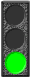Home Page System Simulation About Solar Power FAQ's Easy 5 Step Guide Example Systems Solar Energy Home Solar Projects Solar Combiner Box Mini Junction Box Terms & Definitions Basic Tutorials : Overview Solar Panels Charge Controllers Power Inverters Storage Batteries AC Generators Wires & Cables Meters & Monitors Advanced Info : Watts & Power Solar Radiation Battery Wiring Diagrams Design Tools : System Sizing Estimator Battery Bank Designer Wire Size Calculator Nasa Animations Solar Domain Names Helpful Links Site Map

 Design Tools : System Sizing Estimator
 (Note: These design tools require javascript to be turned on in your browser)
System sizing estimator Quick Start guideI'll figure it out as I go. I want to start clicking buttons!Just give me the short explanation so I can get started.You better explain it to me fairly completely. I want to get it right.
 Brief Instructions for the System Sizing Estimator Use this estimator to get a pretty good idea of how many Solar Panels you will need to generate electricity and how many Storage Batteries you will need to store that electricity. The estimator lets you make simple choices and then calculates the WattHour usage based on typical configurations. After you see how the estimator works, you can figure your own system size much more accurately by determining the wattage needed for each of your appliances and then manually calculating the required WattHours. This procedure is explained under the details of the Estimator's Calculations below. Select closest Size and Use factor for each Item Just click the 'Calculate' button below to see the pre-selected default settings which approximate the typical usage for a small household of 2 persons. Or, select the choices that apply to you from the drop down lists then click the 'Calculate' button below.
System Sizing Estimator
 REFRIGERATORS : Select size in cubic feet. With an icemaker, select the next larger size. 4 to 5 cubic feet 10 cubic feet 12 cubic feet 14 cubic feet 16 cubic feet How many persons are using it? 2 persons is average use (35% run time). NOT USED (1) light use (1-2) below average (2) average use (3) above average (4+) heavy use
 TELEVISIONS : Select a screen size. If it's LCD, select the next smaller size. 9 to 13 inch 19 inch 25 to 27 inch 32 to 36 inch projection TV How many hours per day is the TV on? NOT USED 1 hour or less 2 hours 4 hours 6 hours 8 hours
 MICROWAVE OVENS : Select a MicroWave Oven based on Power rating (Wattage). 600 watts 750 watts 1000 watts 1200 watts 1500 watts How many minutes per day is the MicroWave used? NOT USED 10 minutes 20 minutes 30 minutes 45 minutes 60 minutes
 LIGHTING : Add wattage of lights in all rooms. (four 60 watt bulbs =240 watts) 50 watts 100 watts 150 watts 200 watts 300 watts 400 watts 500 watts How many hours per day are the lights on? NOT USED 1 hour or less 2 hours 3 hours 4 hours 6 hours
 COMPUTERS : LCD displays use less wattage than CRT types. Printers and/or sound systems will increase wattage. Laptop LCD DeskTop LCD DeskTop CRT Sound OR Printer Sound AND Printer How many hours per day is the computer used? NOT USED 1 hour or less 2 hours 3 hours 4 hours 6 hours
 MISCELLANEOUS : This section allows you to add power usage from 25 to 1500 watts for extra items or items not included above. 25 watt device 50 watt device 100 watt device 200 watt device 400 watt device 700 watt device 1000 watt device 1500 watt device How many hours per day is the device used? NOT USED 15 minutes 30 minutes 1 hour 2 hours 4 hours
 * Number of Solar Panels (80-100 watt)  = * Number of Batteries (12v @ 105 AH)  =
Refrigerator watts = WattHours =
Television watts    = WattHours =
MicroWave watts = WattHours =
House lights watts = WattHours =
Computer system = WattHours =
Misc items watts  = WattHours =
Summary of WattHours required
Total daily WattHours required     =
WattHours required for 3 days     =
Battery capacity (50% discharge) =
Battery Bank size in AmpHours
 12 Volt 24 Volt 48 Volt
 * NOTE: The number of Solar Panels required is based on a typical 90 watt panel. The batteries are assumed to be standard 12 volt @ 105 amphours.